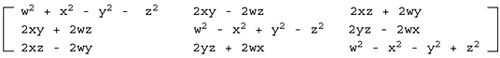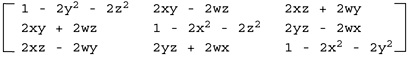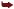# Flight Simulators and Quaternions

Placing your virtual camera inside an airplane cockpit (or a spaceship, for instance) is a bit different from what we have seen so far. It is a first-person camera, but we need to account for six degrees of freedom (three for position, and one each for the roll, pitch, and yaw angles). Besides, most airplanes can perform loops, which imply that the camera is effectively upside down. We must be careful, however. If you take a look at the following equations for the sphere and analyze them, you will discover they are not what we are looking for.

` x = r cos(v pi) cos(u 2 pi) y = r sin(v pi) z = r cos(v pi) sin(u 2 pi) `

Here u would represent yaw, whereas v would represent pitch (forget roll for a second). Now, imagine that we are climbing vertically using such equations to represent movement. The airplane then tries to rotate using the yaw angle to perform any kind of acrobatic move, and unexpectedly, the yaw becomes a roll, and we just roll around the north pole of the parameter sphere.

The problem with the roll, pitch, and yaw representation (sometimes called the Euler angle representation) is that often axes get all mixed up, and combining rotations does not work as expected. This is called the gimbal lock problem. The solution is to use a whole different representation altogether. The representation was devised in the 18th Century by Hamilton and is called quaternions. Quaternions are a powerful mathematical operator that can be used for anything involving orientations, from a flight simulator camera to implementing scripted camera paths. Because it's one of the core techniques used by many games today, I will provide a short tutorial on quaternions.

A quaternion is a mathematical construct that consists of four real numbers. These numbers represent an extension of a complex number. Remember that complex numbers have a real and an imaginary part. Quaternions have one real and three imaginary parts, so their representation is as follows:

` Q = w + xi + yj + zk `

In this representation, (x,y,z) is the vector, and w is the scalar. The vector is not related to regular 3D vectors, so forget about that representation for a second. Quaternions can alternatively be expressed in this form:

` Q = [w,v] `

So, we place the scalar followed by the vector components.

#### Popular Operations

Let's now review how popular operations are implemented using quaternions. Here is the list:

• Addition: q + q' = [w + w', v + v']

• Multiplication: qq' = [ww' - v · v', v * v' + wvv +w'v]

where denotes vector dot product and * denotes vector cross product.

• Conjugate: q* = [w, -v]

• Norm: N(q) = w2 + x2 + y2 + z2

• Inverse: q-1 = q* / N(q)

• Unit quaternion: q is a unit quaternion if N(q)= 1 and then q-1 = q*

• Identity: [1, (0, 0, 0)] (when involving multiplication) and [0, (0, 0, 0)] (when involving addition)

• Rotation of a vector v by a unit quaternion q: v' = q*v*q-1 (where v = [0, v])

Quaternions can be converted to homogeneous matrices and vice versa. To begin with, here is the operation that computes a matrix based on a quaternion:If the quaternion is normalized, we can simplify the preceding transform to the matrix:The opposite operation can also be performed easily. Here is the transform that computes a quaternion based on a Euler rotation:

` q = qyaw qpitch qroll `

where

` qroll = [cos (y/2), (sin(y/2), 0, 0)] qpitch = [cos (q/2), (0, sin(q/2), 0)] qyaw = [cos(f /2), (0, 0, sin(f /2)] `

For completeness, here is the source code for a function that converts a quaternion into a matrix:

` QuatToMatrix(QUAT * quat, float m) { float wx, wy, wz, xx, yy, yz, xy, xz, zz, x2, y2, z2; // calculate coefficients used for building the matrix x2 = quat->x + quat->x; y2 = quat->y + quat->y; z2 = quat->z + quat->z; xx = quat->x * x2; xy = quat->x * y2; xz = quat->x * z2; yy = quat->y * y2; yz = quat->y * z2; zz = quat->z * z2; wx = quat->w * x2; wy = quat->w * y2; wz = quat->w * z2; // fill in matrix positions with them m = 1.0 - (yy + zz); m = xy - wz; m = xz + wy; m = 0.0; m = xy + wz; m = 1.0 - (xx + zz); m = yz - wx; m = 0.0; m = xz - wy; m = yz + wx; m = 1.0 - (xx + yy); m = 0.0; m = 0; m = 0; m = 0; m = 1; } `

And the reverse operation that converts a rotation matrix to its corresponding quaternion follows:

` MatToQuat(float m, QUAT * quat) { float  tr, s, q; int    i, j, k; int nxt = {1, 2, 0}; // compute the trace of the matrix tr = m + m + m; // check if the trace is positive or negative if (tr > 0.0)    {    s = sqrt (tr + 1.0);    quat->w = s / 2.0;    s = 0.5 / s;    quat->x = (m - m) * s;    quat->y = (m - m) * s;    quat->z = (m - m) * s;    } else    {    // trace is negative    i = 0;    if (m > m) i = 1;    if (m > m[i][i]) i = 2;    j = nxt[i];    k = nxt[j];    s = sqrt ((m[i][i] - (m[j][j] + m[k][k])) + 1.0);    q[i] = s * 0.5;    if (s != 0.0) s = 0.5 / s;    q = (m[j][k] - m[k][j]) * s;    q[j] = (m[i][j] + m[j][i]) * s;    q[k] = (m[i][k] + m[k][i]) * s;    quat->x = q;    quat->y = q;    quat->z = q;    quat->w = q;    } } `

Additionally, here is the code for a routine that converts from Euler angles (roll, pitch, and yaw) to quaternion:

` EulerToQuat(float roll, float pitch, float yaw, QUAT * quat) { float cr, cp, cy, sr, sp, sy, cpcy, spsy; // compute all trigonometric values used to compute the quaternion cr = cos(roll/2); cp = cos(pitch/2); cy = cos(yaw/2); sr = sin(roll/2); sp = sin(pitch/2); sy = sin(yaw/2); cpcy = cp * cy; spsy = sp * sy; // combine values to generate the vector and scalar for the quaternion quat->w = cr * cpcy + sr * spsy; quat->x = sr * cpcy - cr * spsy; quat->y = cr * sp * cy + sr * cp * sy; quat->z = cr * cp * sy - sr * sp * cy; } `

So how do quaternions solve our gimbal lock problems? Well, basically, we need to follow a four-step routine.

First, we need to encode rotations using quaternions. If our engine internally uses roll, pitch, and yaw, we can transform those to a quaternion as in the code in the previous section. Second, we will encode frame-to-frame orientation variations in a temporary quaternion, which represents the change in orientation from one frame to the other. Third, we will post-multiply the frame-to-frame quaternion with the original one. This results in a new orientation that combines both rotations. Fourth, we convert the quaternion to a matrix and use matrix multiplication as usual to render the object. By doing so, gimbal lock is gone, and our flight simulator is flying.

Quaternions are not internally supported by OpenGL, so we need to create a new class or library for them. DirectX provides a quaternion object along with all the logical operations in the D3DX utility library. The structure is called D3DXQUATERNION and comes with approximately 20 useful operations, such as the Euler angle-quaternion transform, which can be performed with the following code:

` D3DXQUATERNION*D3DXQuaternionRotationYawPitchRoll(D3DXQUATERNION *pOut, FLOAT Yaw, FLOATPitch, FLOAT Roll); `Core Techniques and Algorithms in Game Programming2003
ISBN: N/A
EAN: N/A
Year: 2004
Pages: 261